# Example of Derivation for a Step-Up/Step-Down Converter – 1

2018.04.19

・Δvout/ΔD and ΔiL/ΔD are derived for each converter.

・Transfer functions are derived in two steps, first considering the stable states of the system, and then determining change amounts for an external disturbance.

・The transfer functions for a step-up/step-down converter are derived by a procedure that is in essence the same as that for a step-up converter or a step-down converter.

We have derived transfer functions for step-down converters and step-up converters. From here, we will embark on derivation of the transfer functions for a step-up/step-down converter. There are a number of control methods used in step-up/step-down converters; here we consider two among these in deriving transfer functions. This time, we focus on the first of these methods.

As we have noted a number of times, the transfer functions for the step-up/step-down converters considered this time are similar to those for step-down and for step-up converters; that is, the transfer functions to be derived are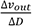and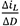, and the transfer functions are similarly derived in two steps.

## Example 1 of Derivation of Transfer Functions for a Step-Up/Step-Down Converter: Step-Up/Step-Down Converter for which ton＝ton’

As stated above, there are various control methods used in step-up/step-down converters. A reason for this is that it is not a single parameter that determines the output voltage for an input voltage. Here we take a moment to explain this briefly.

In the case of a step-down converter, the relation between the input voltage and the output voltage takes the form of the following equation.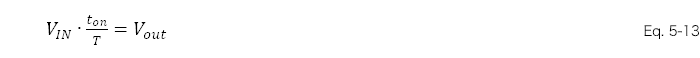As the equation above indicates, if VIN, Vout, and T (the period) are determined for the step-down converter, ton is uniquely determined. However, in the case of a step-up/step-down converter, the following equation obtains, and even if VIN and Vout are determined, there is no unique result.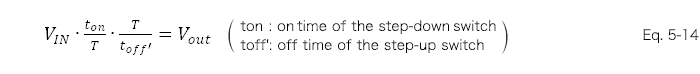Hence there are various control methods. As one of these, in this section we derive the transfer functions for a step-up/step-down converter for which ton＝ton’.

## Step-Up/Down Converters for which ton＝ton’

The circuit on the right uses a control method in which the step-up switch is turned on in sync with the step-down switch. In this case, similarly to the cases of a step-down and a step-up converter, we set up the equations in two steps.

●Step 1: Consider the stable states of the system

①The coil current does not change over one period
②The capacitor charge amount does not change
over one period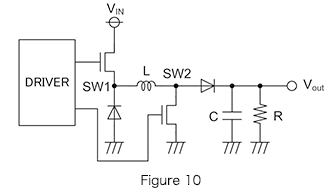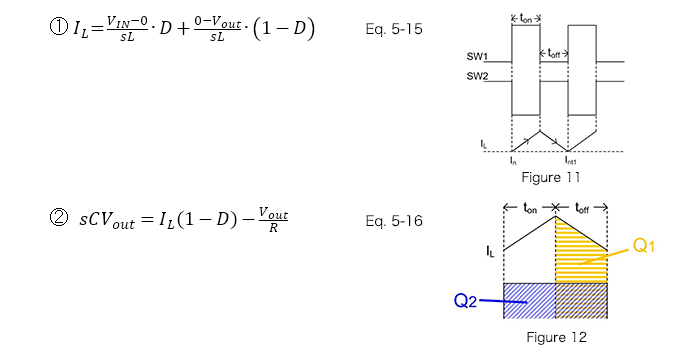●Step 2: Determine change amounts for an external disturbance, and describe the transfer functions

A calculation example from equations 5-15 and 5-16 above is shown below.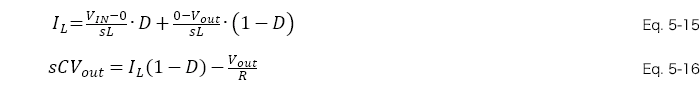Upon substituting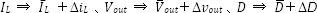in equations 5-15 and 5-16, the following is obtained.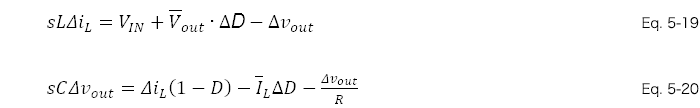Taking equations 5-19 and 5-20 as a system of simultaneous equations and determining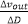and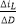, we obtain the following.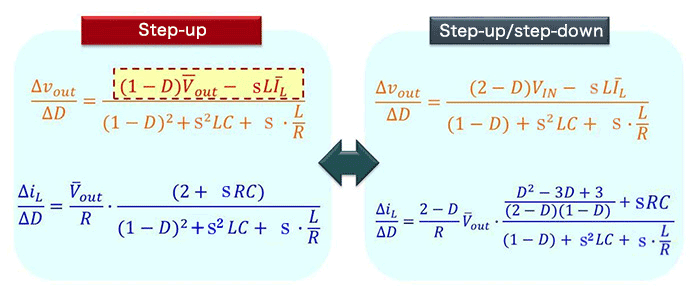Upon comparing with the transfer functions for the step-up converter in the previous section, we see that ultimately, characteristic results similar to those for a step-up converter are obtained.

Next time, we will derive transfer functions for a step-up/step-down converter for which ton≠ton’.